﻿ Experimental Assumption of Steel Pipe Modal Parameters Using Common and Simplified ApproachPublications are Open
Access in this journal
Article Versions
Export Article
• Normal Style
• MLA Style
• APA Style
• Chicago Style
Research Article
Open Access Peer-reviewed

Experimental Assumption of Steel Pipe Modal Parameters Using Common and Simplified Approach

Martin Hagara , Jozef Bocko
American Journal of Mechanical Engineering. 2017, 5(6), 284-288. DOI: 10.12691/ajme-5-6-10
Published online: December 15, 2017

Abstract

The contribution deals with describing of two experimental approaches leading to assumption of modal parameters in a form of natural frequencies, mode shapes and damping of freely supported steel pipe. In the first approach, the model of the pipe was created in common way using planar elements. In the second one, the model of the pipe was simplified using line elements, which should lead to the increasing of time needed for realization of experimental investigation. The results obtained by both approaches as well as their pros and cons are described in the paper.

1. Introduction

There are lot of problems in engineering praxis caused by a fact that mechanical structures work at frequencies, by which a phenomenon well known as resonance is occurred. The resonance increases the noise in the working environment or can even destroy the structure, if the damping elements are not able to absorb the raising magnitudes of vibration, what means that such phenomenon is de facto contrary.

The aim of experimental modal analysis, known as modal testing, is to assume important dynamic characteristics of the structure in a form of natural frequencies, mode shapes and damping, called modal parameters. Its principle consists in the investigation of a relation between the vibration response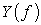and the excitation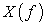at different locations of analyzed structure, known as frequency response function (FRF) 1, 2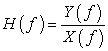(1)

2. Determination of FRF of Systems with One- and Multi-degree of Freedom

Considering mechanical oscillator with viscous damping depicted in Figure 1. According to the second Newton’s law its motion can be expressed by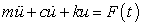(2)

Using Laplace transform eq. (2) leads to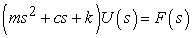(3)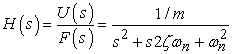(4)

where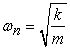is the undamped angular frequency and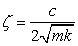is the damping ratio.

• Figure 1. Scheme of analyzed mechanical oscillator

However, by the identification of dynamic system it is not possible to determine the frequency response function due to eq. (4), but it is necessary to find a ratio between the response and excitation spectrum. This function can be expressed using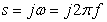in a form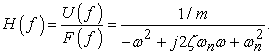(5)

Eq. (5) can be adjusted to more practical form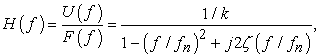(6)

where the denominator is a function of relative frequency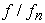.

The mathematical expression of the magnituge as well as the phase shift is given by 3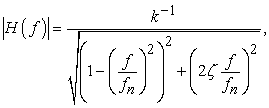(7)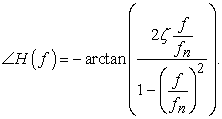(8)

Finding the extreme of eq. (7) leads to the information related to frequency, denoted as damped resonance frequency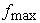due to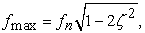(9)

which is valid for the values of damping ratio up to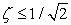. The maximal amount of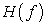magnitude can be expressed by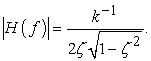(10)

For a reason that the response can be measured in forms of displacement, velocity or acceleration, the frequency response function can also be expressed in different ways (see Table 1)

For the purpose of investigation of multi-degree of freedom (MDoF) system, it is suitable to work with matrices. The equation of motion of the system is then expressed by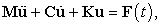(11)

where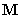is the mass matrix,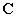is the damping matrix,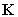is the stiffness matrix and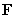is a vector of external forces acting on the system.

Using Laplace transform eq. (11) can be transformed to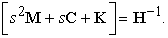(12)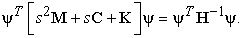(13)

where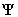is a matrix of mode shapes as well as consideration of proportional damping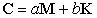(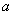,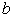are real constant) and the orthogonality of mode shapes, eq. (13) can be described by relation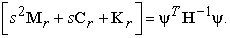(14)

If the inversion of the product of three matrices can be expressed by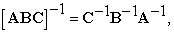after adjustment the frequency response matrix can be described by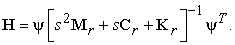(15)

The inverse matrix mentioned in eq. (15) corresponds to the matrix of reciprocal of diagonal elements, denoted as inverse pole matrix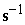5. For each mode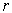, the elements lying on the diagonal of this matrix take the value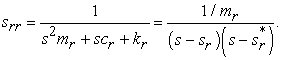(16)

Thereafter, the frequency response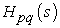can be expressed by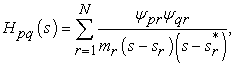(17)

where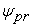and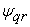are the mode shapes coefficients in locations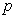and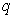for mode.

Considering the residuum in a form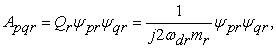(18)

where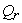is the modal scale factor, the frequency response can be described by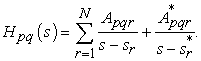(19)

Such relation is valid either for all mode shapes with consideration of proportional damping, or for complex mode shapes with consideration of non-proportional damping.

The residua can be simply modified into matrix form, whereby the residual matrix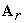has a rank of 1, because it is a linear combination of one vector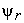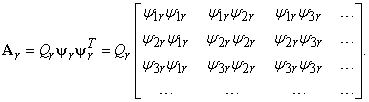(20)

Finally, the overal frequency response function can be expressed in the form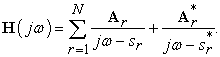(21)

3. Experimental Modal Analysis of the Steel Pipe

The process of assumption of modal parameters through experimental modal analysis consists in the following steps:

• the choice of type and amount of excitation and measuring devices,

• the creation of the analyzed structure geometry,

• the determination of degrees of freedom (locations of excitation, or measuring of response),

• the setting of frequency range, the accuracy of the measurement and averaging,

• the trigger,

• the choice of weighting function,

• the realization of the measurements.

For a reason that the measurements realized in particular locations at analyzed structure are commonly repeated and the results averaged, the determination of convenient number of degrees of freedom is a task, which mainly affects the duration of modal parameters assumption. This paper deals with the determination of the influence of the degrees of freedom number on the values of natural frequencies, damping and mode shapes of a freely supported steel pipe. The length of the pipe was equal to 900 mm, its diameter 120 mm and thickness 1 mm. Free support of the pipe was realized by its hanging to the robust construction through soft rubber band (see Figure 2).

• Figure 2. Free support of the analyzed pipe realized by soft rubber band

Two experiments were realized with the aim to compare the results obtained by the use of full (planar) model and the simplified (line) model of the pipe. Therefore, the excitation was realized in 88 locations (Figure 3a) or 18 points (Figure 3b) using the impact hammer Brüel & Kjær 8206. The response was in both approaches measured by 4 accelerometers Brüel & Kjær 4507B, attached in the same positions of the pipe.

• Figure 3. Models of geometry: a) full (planar), b) simplified (line)

The frequency range of the measurement was set from 0 Hz up to 1.6 kHz. The number of spectral lines was set to 800, what means that the accuracy of the measurement was equal to 2 Hz and the shutter time was 500 ms. Each degree of freedom was excited three-times and the results were linearly averaged.

• Figure 4. CMIF function acquired from the full model
• Figure 5. CMIF function obtained from the simplified model

After the settings of trigger and weighting, both approaches of assumption of steel pipe modal parameters were realized. The complex mode indicator functions (CMIF) acquired in PULSE Reflex® software from both measurements are depicted in Figure 4 and Figure 5.

Using the Rational Fraction Polynomial-Z with 40 iterations as modal parameters estimation method for both approaches, the PULSE Reflex® software assumed the damped natural frequencies (DNF) and damping (D) present in Table 2.

Modes denoted as 2a, 2b up to 7a, 7b are the coupled modes, incident mainly by axially symmetric structures. As can be seen from Table 2. the eleventh mode together with the fifth as well as the sixth coupled mode were not found by performing of simplified investigation. On the other hand, the natural frequencies and damping acquired by both approaches reach approximately the same values.

The comparison of corresponding mode shapes is present in Table 3.

4. Summary

The paper describes two approaches how to perform experimental modal analysis of steel pipe. In the first one, the full model of the pipe was created, the second (simplified) model was considered as a line one. Whereas in the first investigation it was necessary to excite the model in 88 locations, in the second one in only 18 locations. The duration of the modal testing increased approximately five-times, however the estimated natural frequencies as well as damping were achieved nearly the same values. In simplified approach, three modes estimated by full model, were not found. Using simplified model it was not easy to characterize some coupled mode shapes. Finally, it can be stated, that experimental modal analysis can be realized in simplified approach, but only with the acceptance of some compromises mainly in the way of mode shapes depicting.

Acknowledgements

The paper is the result of the projects implementation VEGA 1/0731/16 and VEGA 1/0393/14.

References

  He, J. and Fu, Z.-F., Modal Analysis, Great Britain, 2001. In article  Inman, D.J., Vibration with Control, John Wiley and Sons, Ltd., 2006. In article View Article  Bilošová A., Aplikovaný mechanik jako součást týmu konstruktérů a vývojárů: Část MODÁLNÍ ZKOUŠKY, VŠB – TU Ostrava, 2012. In article  Miláček, S., Modální analýza mechanických kmitů, ČVTU v Praze, 1992. In article  Brandt A., Noise and Vibration Analysis: Signal Analysis and Experimental Procedures, John Wiley and Sons, Ltd., 2011. In article View ArticleThis work is licensed under a Creative Commons Attribution 4.0 International License. To view a copy of this license, visit http://creativecommons.org/licenses/by/4.0/